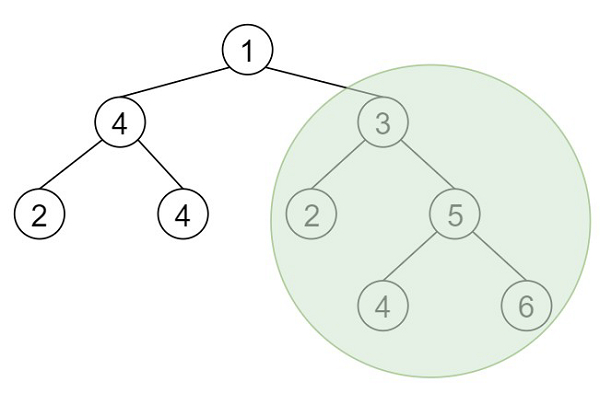# Maximum Sum BST in Binary Tree in C++

Suppose we have a binary tree root, we have to find the maximum sum of all nodes of any subtree which is also a Binary Search Tree (BST).

So, if the input is like,then the output will be 20, this is the sum of all nodes in the selected BST.

To solve this, we will follow these steps −

• Create one block called Data, this will hold some members like sz, maxVal, minVal, ok, sum. Also define one initializer for data, that will take in this order (sz, minVal, maxVal, ok, and set sum as 0)

• ret := 0

• Define a method called solve(), this will take tree root

• if not node is non-zero or val of node is same as 0, then −

• return a new data with initializing it by (0, inf, -inf, true)

• left := solve(left of node)

• right = solve(right of node)

• Create one Data type instance called curr

• curr.ok := false

• if node - > val >= right.minVal, then −

• return curr

• if node - > val <= left.maxVal, then −

• return curr

• if left.ok is non-zero and right.ok is non-zero, then −

• curr.sum := val + left.sum + right.sum of node

• ret := maximum of curr.sum and ret

• curr.sz := 1 + left.sz + right.sz

• curr.ok := true

• curr.maxVal := maximum of node value and right.maxVal

• curr.minVal := maximum of node value and left.minVal

• return curr

• From the main method do the following

• ret := 0

• solve(root)

• return ret

Let us see the following implementation to get better understanding −

## Example

Live Demo

#include <bits/stdc++.h>
using namespace std;
class TreeNode{
public:
int val;
TreeNode *left, *right;
TreeNode(int data){
val = data;
left = NULL;
right = NULL;
}
};
void insert(TreeNode **root, int val){
queue<TreeNode*> q;
q.push(*root);
while(q.size()){
TreeNode *temp = q.front();
q.pop();
if(!temp->left){
if(val != NULL)
temp->left = new TreeNode(val);
else
temp->left = new TreeNode(0);
return;
}else{
q.push(temp->left);
}
if(!temp->right){
if(val != NULL)
temp->right = new TreeNode(val);
else
temp->right = new TreeNode(0);
return;
}else{
q.push(temp->right);
}
}
}
TreeNode *make_tree(vector<int> v){
TreeNode *root = new TreeNode(v);
for(int i = 1; i<v.size(); i++){
insert(&root, v[i]);
} return root;
}
struct Data{
int sz;
int maxVal;
int minVal;
bool ok;
int sum;
Data(){}
Data(int a, int b, int c, bool d){
sz = a;
minVal = b;
maxVal = c;
ok = d;
sum = 0;
}
};
class Solution {
public:
int ret = 0;
Data solve(TreeNode* node){
if (!node || node->val == 0)
return Data(0, INT_MAX, INT_MIN, true);
Data left = solve(node->left);
Data right = solve(node->right);
Data curr;
curr.ok = false;
if (node->val >= right.minVal) {
return curr;
}
if (node->val <= left.maxVal) {
return curr;
}
if (left.ok && right.ok) {
curr.sum = node->val + left.sum + right.sum;
ret = max(curr.sum, ret);
curr.sz = 1 + left.sz + right.sz;
curr.ok = true;
curr.maxVal = max(node->val, right.maxVal);
curr.minVal = min(node->val, left.minVal);
}
return curr;
}
int maxSumBST(TreeNode* root){
ret = 0;
solve(root);
return ret;
}
};
main(){
Solution ob;
vector<int> v =
{1,4,3,2,4,2,5,NULL,NULL,NULL,NULL,NULL,NULL,4,6};
TreeNode *root = make_tree(v);
cout << (ob.maxSumBST(root));
}

## Input

{1,4,3,2,4,2,5,NULL,NULL,NULL,NULL,NULL,NULL,4,6}

## Output

20